# GED Math : Pie Charts

## Example Questions

1 3 Next →

### Example Question #21 : Representing Data

The following graph shows the distribution of students at a high school that has a student population of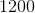: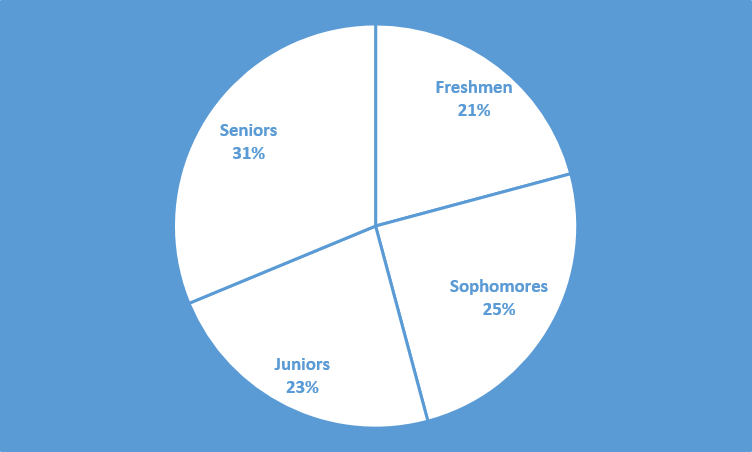How many freshmen does this school have?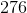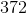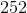Explanation:From the chart, we can see that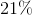of the school population is freshmen. Thus, we will need to findof.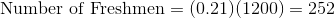### Example Question #22 : Representing Data

The following graph shows the distribution of students in a high school that has a student population of:In degrees, what is the central angle of the sector labeled "Juniors"? Round to the nearest tenths place.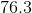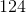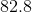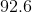Explanation:The sector labeled "Juniors" takes up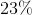of the circle. Thus, its central angle must beof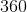, the number of total angles in a circle.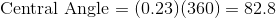The central angle must bedegrees.

### Example Question #23 : Representing Data

The following graph shows the distribution of students in a high school that has a student population of:How many more juniors and seniors are there than freshmen and sophomores?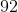Explanation:The juniors and seniors make up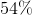of the school's population. Thus, we can find the number of juniors and seniors.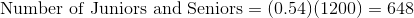The freshmen and sophomores make up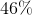of the school's population. Thus, we can find the number of freshmen and sophomores.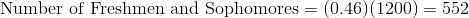Subtract these two numbers to find how many more juniors and seniors there are than freshmen and sophomores.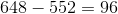There aremore juniors and seniors than there are freshmen and sophomores.

1 3 Next →